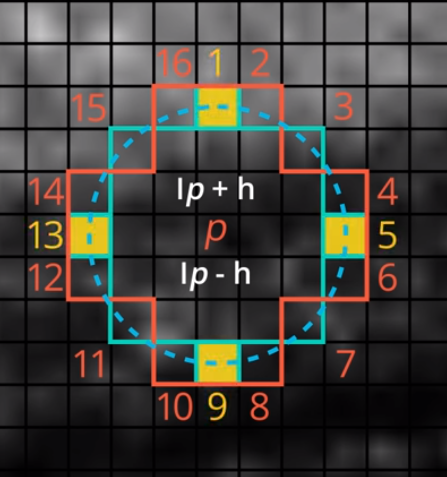# Understanding Keypoints and ORB algorithm

Source: Deep Learning on Medium## Keypoint in detail

A keypoint is calculated by considering an area of certain pixel intensities around it. Keypoints are calculated using various different algorithms, ORB technique uses the FAST algorithm to calculate the keypoints. FAST stands for Features from Accelerated Segments Test. FAST calculates keypoints by considering pixel brightness around a given area. Consider a pixel area in an image and lets test if a sample pixel p becomes a keypoint

Considering an area of 16 pixels around the pixel p. In the image, the intensity of pixel p is represented as ip and predefined threshold as h. A pixel is brighter if the brightness of it is higher than ip+h and it is of lower brightness if its brightness falls lower than ip-h and the pixel is considered the same brightness if its brightness is in the range ip+h and ip-h.

FAST decides the point p as keypoint if at least 8 pixels have higher brightness than the pixel p in 16 pixels intensities marked in a circle around it or the same result can be achieved by comparing 4 equidistant pixels on the circle i.e., pixels 1,5,9 and 13. This reduces the time taken to calculate keypoints by 4 times

Keypoints provide us the locations where the pixel intensities are varying. We get the prominent corners of an object from which we can identify an object from opposed to any other object in an image.